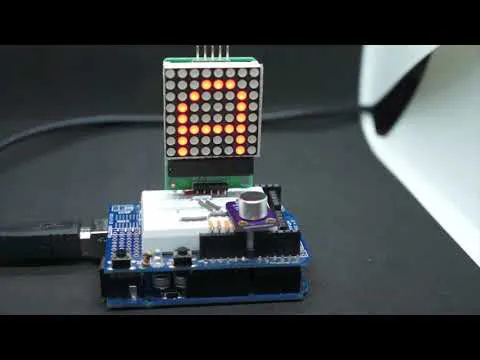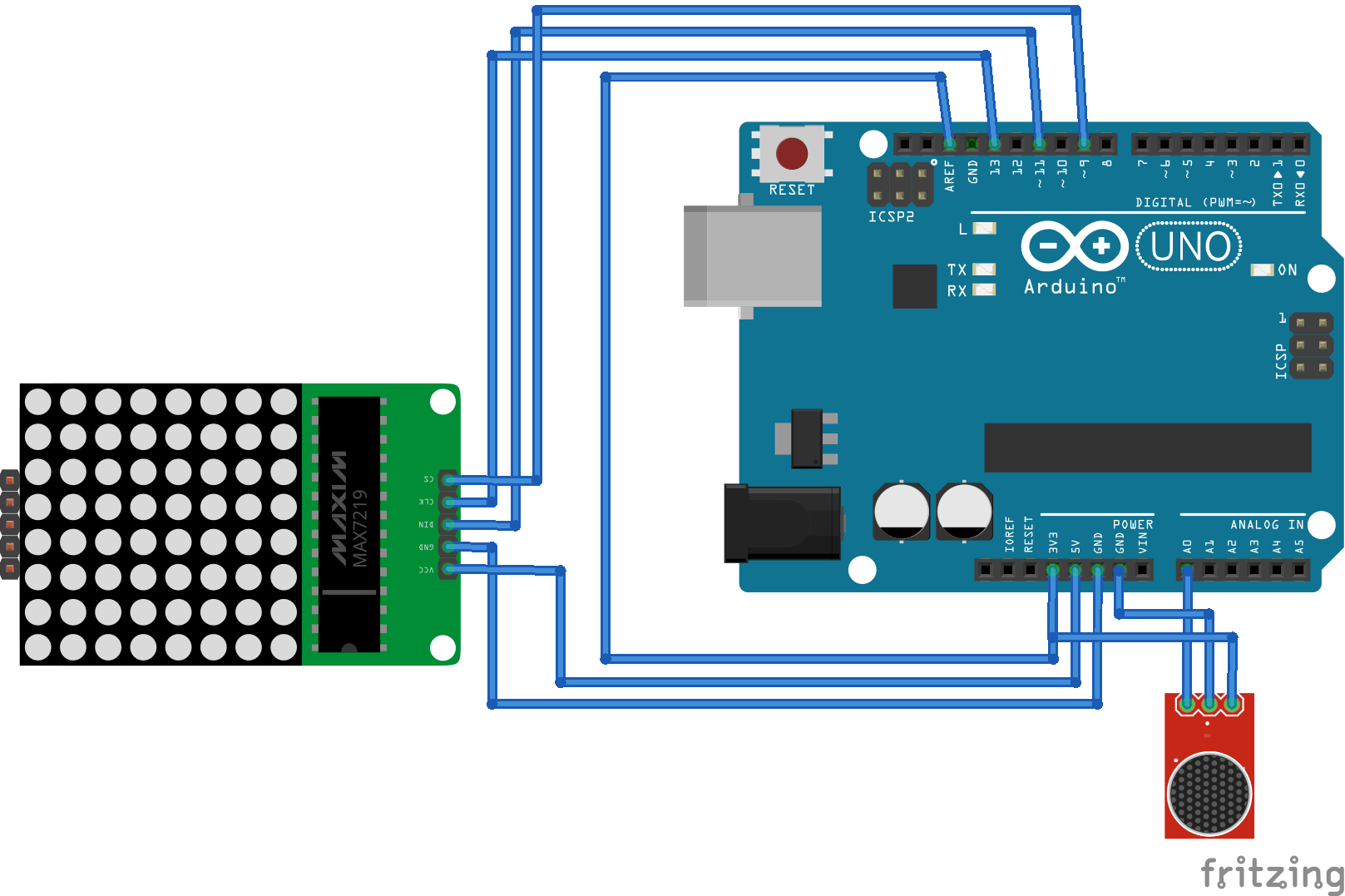Detecting DTMF encoded digit by capturing mic input with an Arduino Uno.

• 5,870 views
• 7 respects

## Components and supplies

I was inspired to build this device by a home assignment on Digital Signal Processing online course. This is a DTMF decoder implemented with Arduino Uno and in this article I’ll explain how it works.

In DTMF each symbol is encoded with two frequencies according to the table

The device captures input from the microphone and calculates amplitudes of eight frequencies for further analysis. Let’s consider the algorithm in greater details.

### Data acquisition

In order to perform spectrum analysis samples should be captured at a certain predictable frequency. To achieve this I used free-run ADC mode with maximum precision (prescaler 128) it gives sampling rate 9615Hz. The code below shows how to configure Arduino’s ADC.

``````void initADC() {
// Init ADC; f = ( 16MHz/prescaler ) / 13 cycles/conversion
ADCSRB = 0; // Free-run mode
DIDR0  = _BV(0); // Turn off digital input for ADC pin
TIMSK0 = 0;                // Timer0 off
}
``````

And the interrupt handler looks like this

``````ISR(ADC_vect) {
uint16_t sample = ADC;samples[samplePos++] = sample - 400;

if(samplePos >= N) {
}
}
``````

### Spectrum analysis

After collecting samples I calculate amplitudes of 8 frequencies encoding symbols. I don’t need to run full FFT for this, so I used Goertzel’s algorithm.

``````void goertzel(uint8_t *samples, float *spectrum) {
float v_0, v_1, v_2;
float re, im, amp;

for (uint8_t k = 0; k < IX_LEN; k++) {

float a = 2. * c;
v_0 = v_1 = v_2 = 0;
for (uint16_t i = 0; i < N; i++) {
v_0 = v_1;
v_1 = v_2;
v_2 = (float)(samples[i]) + a * v_1 - v_0;
}
re = c * v_2 - v_1;
im = s * v_2;
amp = sqrt(re * re + im * im);
spectrum[k] = amp;
}
}
``````

This is how digit 3 looks like encoded with DTMF

The rest of the code is pretty straightforward, full code can be found here. Let’s move on to building the device.

### Schematics

I used the following components:

The video below shows how it works

### Conclusion

What could be improved here? I used N = 256 samples at rate 9615Hz which has some spectrum leakage, if N = 205 and rate is 8000Hz then the desired frequencies coincide with discretisation grid. For that ADC should be used in timer overflow mode.

Source article

## Code

##### Code snippet #1Plain text
```void initADC() {
// Init ADC; f = ( 16MHz/prescaler ) / 13 cycles/conversion
ADCSRB = 0; // Free-run mode
DIDR0  = _BV(0); // Turn off digital input for ADC pin
TIMSK0 = 0;                // Timer0 off
}
```
##### Code snippet #2Plain text
```ISR(ADC_vect) {
uint16_t sample = ADC;samples[samplePos++] = sample - 400;

if(samplePos >= N) {
}
}
```
##### Code snippet #3Plain text
```void goertzel(uint8_t *samples, float *spectrum) {
float v_0, v_1, v_2;
float re, im, amp;

for (uint8_t k = 0; k < IX_LEN; k++) {

float a = 2. * c;
v_0 = v_1 = v_2 = 0;
for (uint16_t i = 0; i < N; i++) {
v_0 = v_1;
v_1 = v_2;
v_2 = (float)(samples[i]) + a * v_1 - v_0;
}
re = c * v_2 - v_1;
im = s * v_2;
amp = sqrt(re * re + im * im);
spectrum[k] = amp;
}
}
```

## Schematics#### Published on

December 23, 2018

#### Members who respect this project

See similar projects
you might like

#### Automation basics using arduino and DTMF decoder

Project tutorial by TEAM DIY

• 4,995 views
• 7 respects

#### DTMF Decoder Using Only Arduino

Project tutorial by MM_Shoaib

• 7,574 views
• 1 comment
• 5 respects

• 2,710 views
• 6 respects

#### Adaptive LED Morse Code Decoder and Timer Interrupt

Project tutorial by shjin

• 3,322 views
• 1 comment
• 16 respects

#### Generate DTMF Tones Only Using Arduino

Project tutorial by Dilshan Jayakody

• 5,299 views
• 1 comment
• 6 respects

• 19,976 views
• 1 comment
• 31 respects